can someone please help me figure out these 5 questions with the calculations shown please. and i need help with the graph ??Acid-Base Titration of a Weak Acid with a Strong Base: Determination of K. Introduction: You will be titrating a solution of a weak acid with 0.100 M NaOH, while monitoring the reaction using a pH meter. Weak acids have characteristic acid-ionization constants, K. The purpose of this lab is to use the titration to determine the value of this constant for the weak acid called “benzoic acid” (HC-H502). The reaction equation is below: HC,H,O2 + NaOH NaC-H502 + H2O The pH curve for titration of acetic acid (a weak acid related to benzoic acid) is shown below. It differs from the pH curve for a strong acid in a few key ways. pH Curve for Titration of HC HO with NaOH 2 3 2 14.00 12.00 ocas 10.00 рн 3Fquivalence Pont 8.00 Haway Point foH = akaj 6.00 eeee 4.00 998 2.00 000 000 40 on 5000 2000 1000 Wu une ol Nai Because the titration involves the creation of a salt of a weak acid, the salt itself acts as a weak base. So, unlike the titration of a strong acid, the "neutralization point" in the titration occurs at a basic pH. (The term "equivalence point" is sometimes used since the salt solution will not have a neutral pH of 7.) The equivalence point (and equivalence pH) of the titration can be determined from the pH curve by finding the curve's point of inflection. This will always correspond to the equivalence point, just as it does for titration of a strong acid.
For titration of a weak acid, the pH curve also provides a way of determining the value of the acid ionization constant. At the point on the curve corresponding to one-half of the volume of base needed to reach the equivalence point, the pH of the solution will equal the pKa oft of the acid. The pK, is related to K, the way pH is related to hydrogen ion concentration: pKa = -log K OR K. =antilog(-pK) Example: If the p for a weak acid is 3.56, then K. - antilog (-3.56) - 2.8 x 10-4.
Acid-Base PH Report Sheet1 Total volume of Base Added pH Total volume of Base Added pH 3.27 8.0 11.99 1 3.71 9.0 12.08 2 4.15 12.15 10.0 15.0 2.5 4.32 12.34 3 4.51 3.25 4.58 3.5 4.72 4.93 I 3.75 4.0 5.13 4.1 5.24 4.2 5.38 4.3 5.57 5.88 4.6 6.86 4.7 9.54 4.8 10.30 4.9 10.79 5.0 10.90 5.2 11.08 5.3 11.19 5.4 11.28 5.5 11.35 5.75 11.46 6.0 11.57 6.5 11.74 7.0 11.85
Acid-Base pH Titration of a Weak Acid with a Strong Base Report Sheet 2 Questions 1. What volume of base was required to reach the equivalence point? 2. What is the volume at the halfway point to equivalence? 3. What is the pH at the halfway point? I 4. The answer to question 3 is also the p. of benzoic acid. Calculate the value for K. 5. Salicylic acid (HC H:03) is closely related to benzoic acid in structure, and has a p. of 2.98. Considering your data for this experiment, which is the stronger acid, benzoic acid or salicylic acid? (Hint: Think about the relationship between acid strength and K.)
pH Titration Curve for Benzoic Acid/NaOH 140 130 100 B. pH 2 D 19 110 150 Volume of NaOH (mL)

We need 10 more requests to produce the answer to this homework help question. Share with your friends to get the answer faster!

0 /10 have requested the answer to this homework help question.

Once 10 people have made a request, the answer to this question will be available in 1-2 days.
All students who have requested the answer will be notified once they are available.

#### Earn Coin

Coins can be redeemed for fabulous gifts.

Similar Homework Help Questions
• ### can someone help me answer these 5 questions and figire this graph out please? Acid-Base Titration...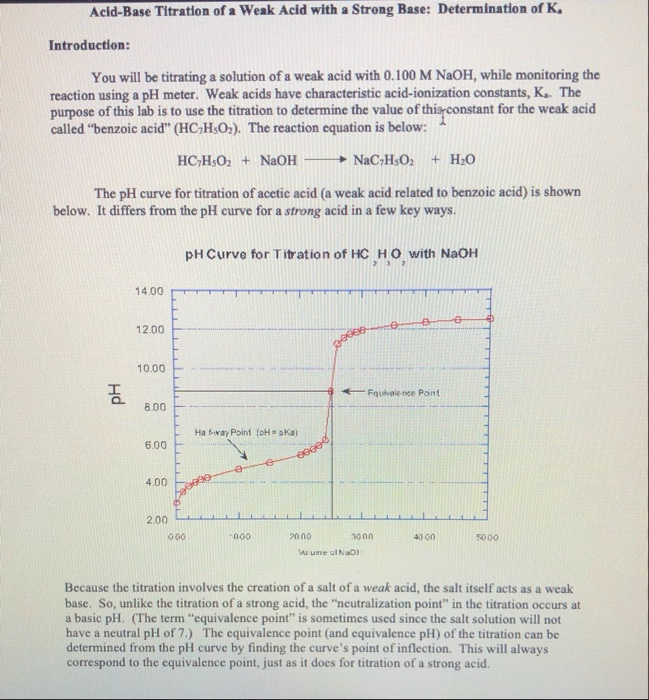can someone help me answer these 5 questions and figire this graph out please? Acid-Base Titration of a Weak Acid with a Strong Base: Determination of K. Introduction: You will be titrating a solution of a weak acid with 0.100 M NaOH, while monitoring the reaction using a pH meter. Weak acids have characteristic acid-ionization constants, K. The purpose of this lab is to use the titration to determine the value of this constant for the weak acid called “benzoic...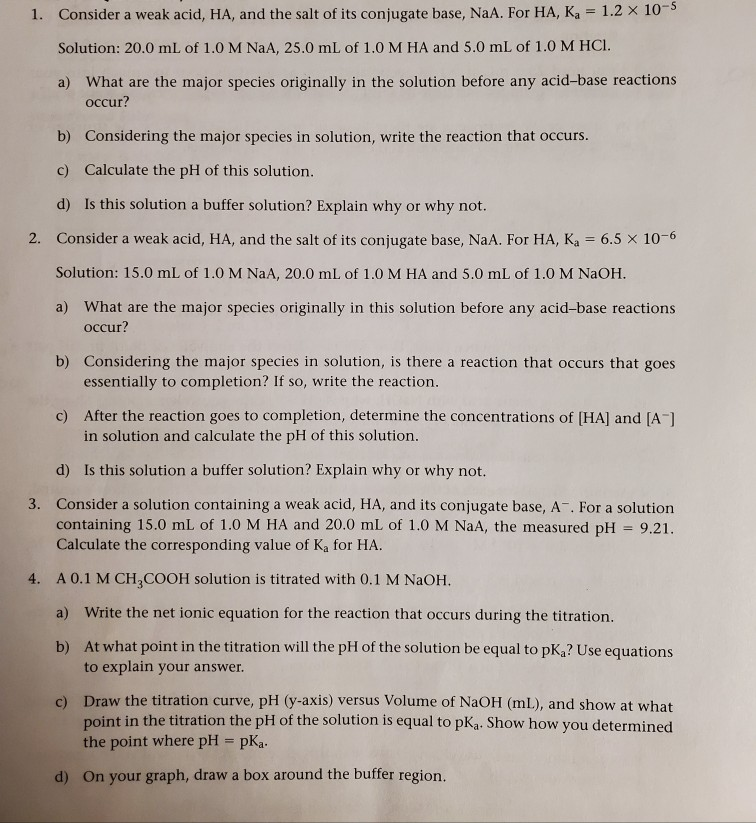can someone please help me out with questions 1-5, please To add more information this was given to me for a lab that used a weak acid and we added a strong base through titration. We just observed how the ph changes. Later we then used a buffer with a weak acid to see how buffers affect ph change. These questions are basically surrounded around those topics to help us prepare. However, I'm kinda confused about answering them because weak...

• ### Answer the questions using the data and graph. please show all work. Thank you Titration of...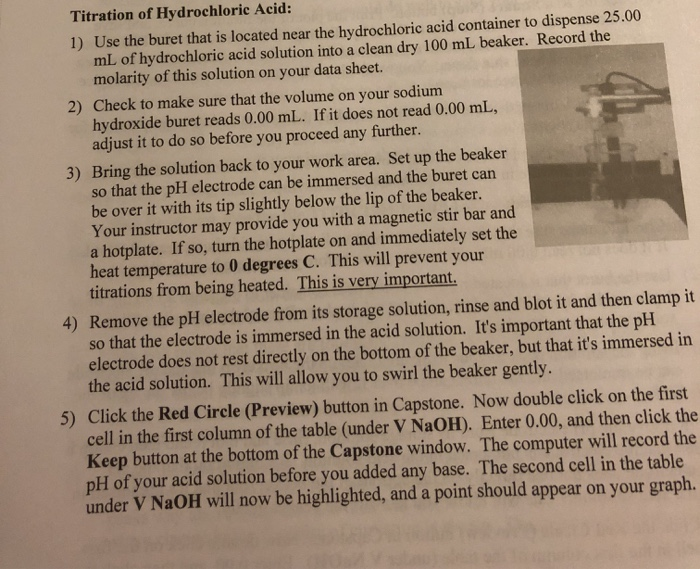Answer the questions using the data and graph. please show all work. Thank you Titration of Hydrochloric Acid: 1) Use the buret that is located near the hydrochloric acid container to dispense 25.00 mL of hydrochloric acid solution into a clean dry 100 mL beaker. Record the molarity of this solution on your data sheet. 2) Check to make sure that the volume on your sodium hydroxide buret reads 0.00 mL. If it does not read 0.00 mL, adjust it...

• ### In the second image it states that after I pass the equivalence point, I should add...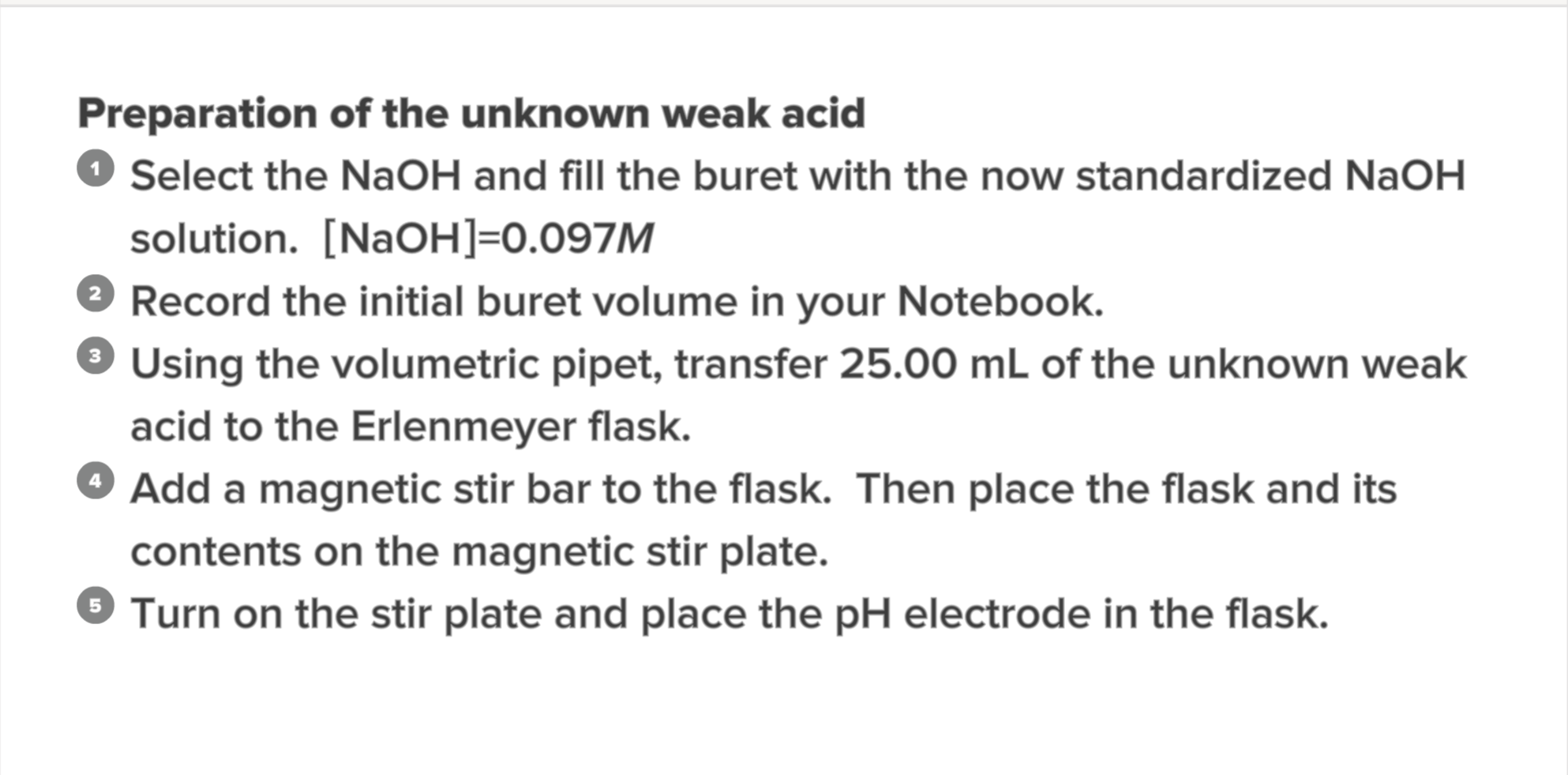In the second image it states that after I pass the equivalence point, I should add 2ml of aliquots of NaOH until there's no PH change. How do I identify when the equivalence point is? Preparation of the unknown weak acid Select the NaOH and fill the buret with the now standardized NaOH solution. [NaOH]=0.097M Record the initial buret volume in your Notebook. 3 Using the volumetric pipet, transfer 25.00 mL of the unknown weak acid to the Erlenmeyer flask....

• ### Please help me with the titration curve diagram questions. Number 22 and 23. If you could...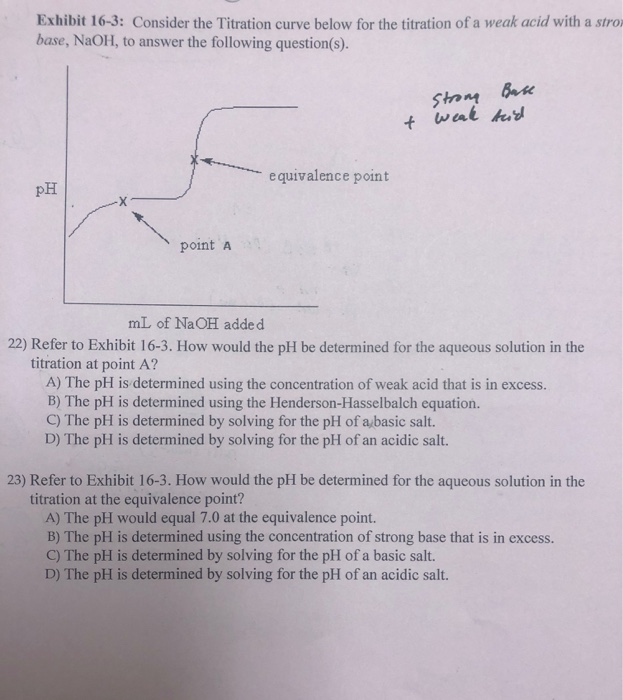Please help me with the titration curve diagram questions. Number 22 and 23. If you could also explain to me how you found the answer to the problem. Thank you! Exhibit 16-3: Consider the Titration curve below for the titration of a weak acid with a stro base, NaOH, to answer the following question(s). Strong t weak Base tid equivalence point PH point a mL of NaOH added 22) Refer to Exhibit 16-3. How would the pH be determined for...

• ### Need help with 3 and 4 on calculations. some info is on top of the page...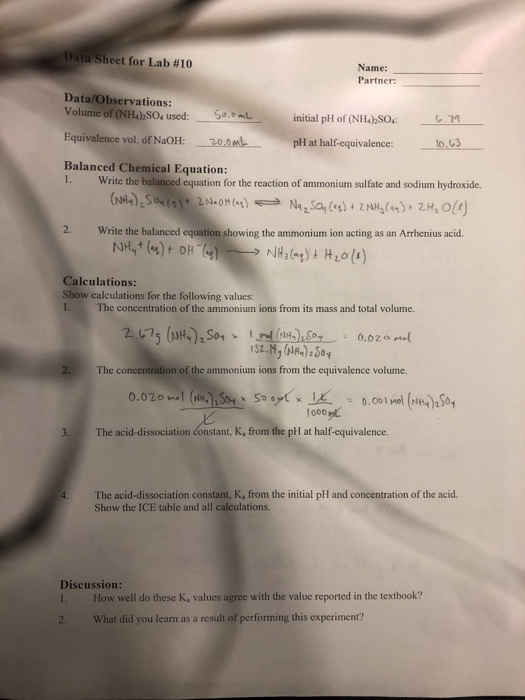Need help with 3 and 4 on calculations. some info is on top of the page Data Sheet for Lab #10 Name: Partner: Data/Observations: Volume of (NH), SO, used: Suomi initial pH of (NH4)2SO4 6.79 Equivalence vol. of NaOH: 20.00 pH at half-equivalence: 10,63 Balanced Chemical Equation: 1. Write the balanced equation for the reaction of ammonium sulfate and sodium hydroxide. (NH4)2SO4(s)? 2 N.0H(1) Nasa, () + 2NH3(-) + 2H2O 2. Write the balanced equation showing the ammonium ion acting...

• ### Consider the titration of 40.0 mL of 0.500 M NH, with 1.00 M HC a) What...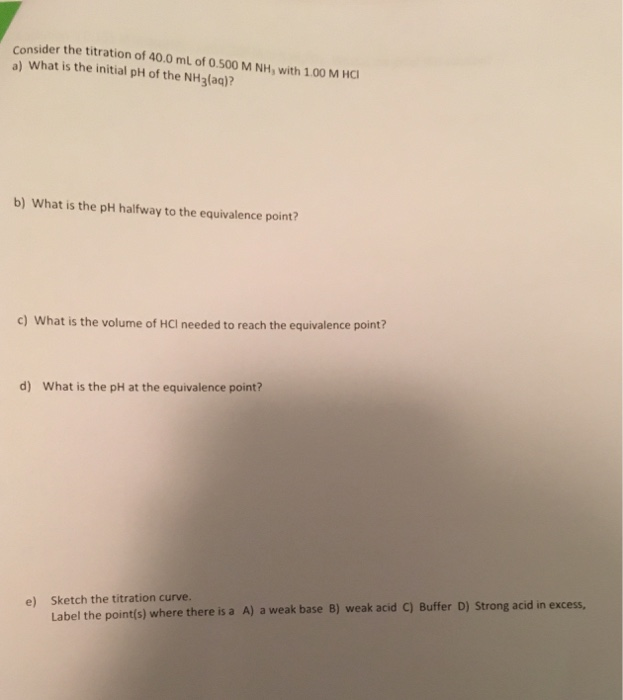Consider the titration of 40.0 mL of 0.500 M NH, with 1.00 M HC a) What is the initial pH of the NHg(aq)? b) What is the pH halfway to the equivalence point? c) What is the volume of HCI needed to reach the equivalence point? What is the pH at the equivalence point? d) Sketch the titration curve. Label the point(s) where there is a A) a weak base B) weak acid C) Buffer D) Strong acid in excess,...

• ### Equivalence Point for Titration #1: 24.96 mL Equivalence Point for Titration #2: 25.40 mL Equivalence Point...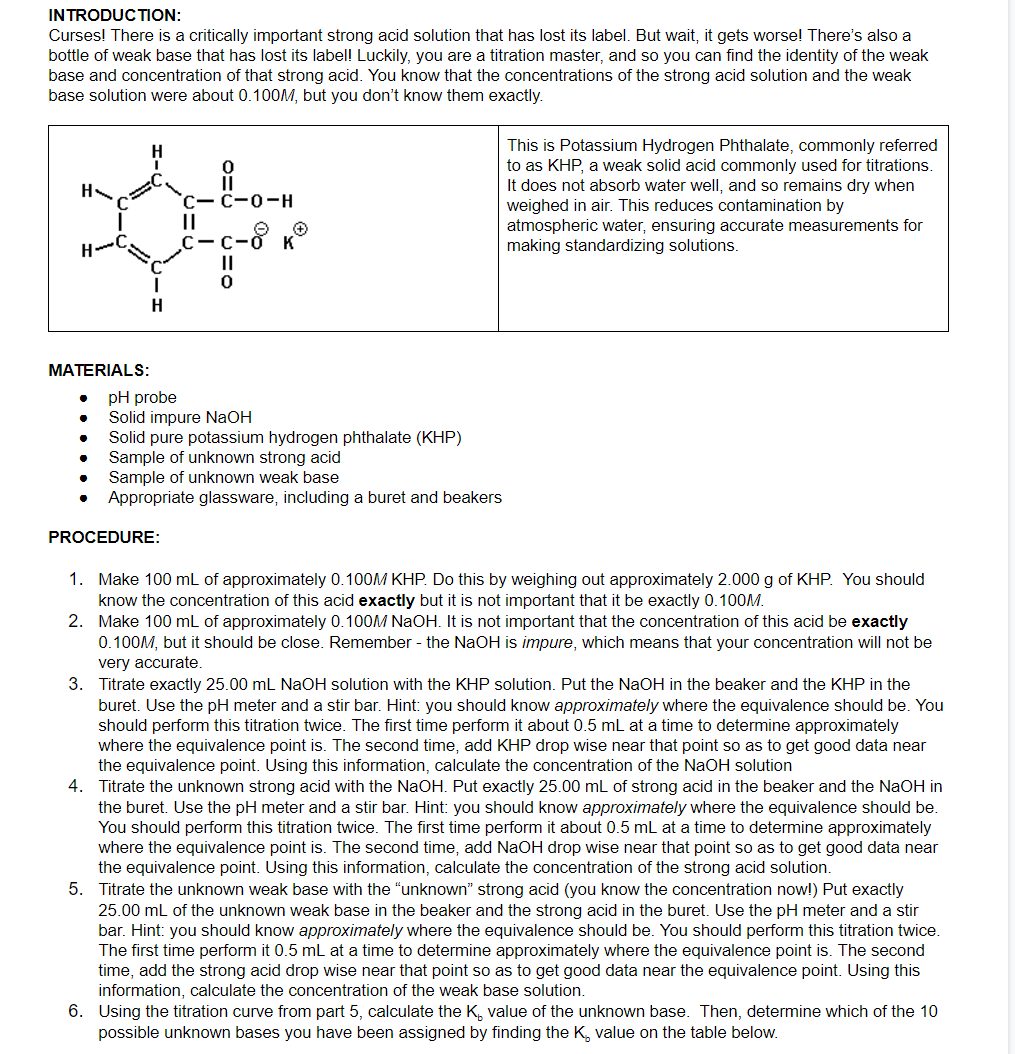Equivalence Point for Titration #1: 24.96 mL Equivalence Point for Titration #2: 25.40 mL Equivalence Point for Titration #3: 25.20 mL Midpoint pH for Titration #3: 9.80 QUESTIONS: 4) Set up the calculation required to determine the concentration of the NaOH solution via titration of a given amount of KHP. Include all numbers except the given mass of KHP. 5) Set up the calculation required to determine the concentration of the unknown strong acid via titration with a known volume...

• ### can someone please me with checking our work for the chart and helping me with the missing ones. Also with question 4 a...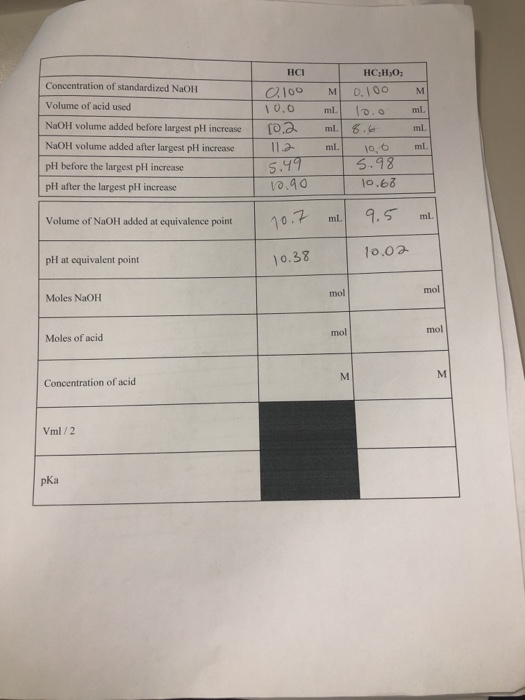can someone please me with checking our work for the chart and helping me with the missing ones. Also with question 4 and 5? Thank You Concentration of standardized NaOH Volume of acid used NaOH volume added before largest pH increase NaOH volume added after largest pH increase pH before the largest pll increase pH after the largest pH increase I HC HC,H,O, 0.100 M 0.100 M 10.0 ml. 10 ml 10.2 ml. 8.6 ml. 112 ml 100 ml 5.495...

• ### Titration of weak acid with strong base pre-lab Answers can only be entered/graded between 2019-10-24 and...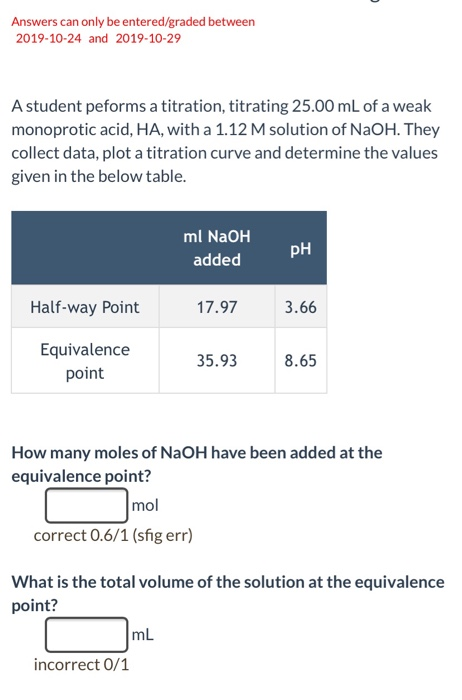Titration of weak acid with strong base pre-lab Answers can only be entered/graded between 2019-10-24 and 2019-10-29 A student peforms a titration, titrating 25.00 mL of a weak monoprotic acid, HA, with a 1.12 M solution of NaOH. They collect data, plot a titration curve and determine the values given in the below table. ml NaOH PH added Half-way Point 17.97 3.66 Equivalence point 35.938.65 How many moles of NaOH have been added at the equivalence point? r mol correct...

Free Homework App